# NET AREA & PERMISSIBLE STRESSES FOR TENSION MEMBERS

NET AREA CALCULATION FOR TENSION MEMBERS

When tension members are spliced or connected to a gusset plate by rivets or bolts, some material is removed from the cross-section due to bolt or rivet holes. The net area at any section is equal to the gross area minus the deduction for holes at that section. The deduction for the hole is the product of the hole diameter and the thickness of the material.

Net effective width,

Where, s = the staggered pitch, i.e. the distance between any two consecutive rivets, in a zigzag chain measured parallel to the direction of stress in the member,

g = gauge distance

n = number of rivet holes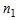= number of zigzags or inclined lines.

This method can be applied to the angles also in which the rows of the rivets in both legs are staggered with respect to each other. For angles, the gross width shall be the sum of the width of the legs less than the thickness. The gauge for holes in opposite legs shall be the sum of gauges from back of angles less the thickness.

#### Net Effective Section for Angles and Tees in Tension

An angle is usually connected to a gusset plate by one leg and a tee is connected through its flange only.

Figure: Tension Members

CASE – 1

In the case of single angles in tension, connected by one leg only,

Net effective area =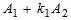Where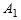is the area of the connected leg,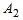= area of the outstanding leg, and

For angle sections, a x b x t,

Where lug angles are used, the net sectional area of the whole angle member could be considered.

CASE – 2

In the case of pair of angles back to back (or a single tee) in tension connected by only one leg on each angle (or by the flange of a tee) to the same side of the gusset plate,

Net effective area =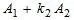Whereis the area of the connected legs (or flange of the tee)= area of the outstanding leg (or web of the tee), and

CASE – 3

In the case of double angles or tees carrying direct tension, placed back to back and connected to both sides of the gusset,

Net effective area = gross area of section – area of holes